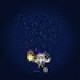2018 Grade 10 Math Challenge Questions 26-50 (with PDF)

This is the 2018 MTAP Grade 10 Math Challenge questions 26 to 30. Questions 1-25 including can be found here. The pdf can be downloaded here. Solutions and answer will be posted later. More reviewers can be found on the Past Tests and All Posts pages.

Questions

26.) Find the radius of the circle with equation$x^2 + y^2 + 4x - 3y + 5 = 0$.

27.) Give the equation (in center-radius form) of the circle having as a diameter the segment with endpoints$(-2, 10)$ and$(4, 2)$.

28.) The center of a circle is on the x-axis. If the circle passes through$(0,5)$ and$(6,4)$, find the coordinates of its center.

29.) If$\theta$ is an angle in a triangle and$\sec \theta = \frac{7}{5}$, find$\tan \theta$

30.) In$\triangle ABC$,$BC = 6$,$CA = 7$, and$AB = 8$. Find$\dfrac{\sin A + \sin C}{ \sin C}$.

31.) In$\triangle ABC$,$AB = 3$,$AC = 2\sqrt{3}$, and$\angle A = 30^\circ$. Find$BC$.

32.) In a circle, chord$PQ$ is bisected by chord$RS$ at$T$. If$RT = 3$ and$ST = 6$, find$PQ$.

33.) Pentagon$ABCDE$ is inscribed in a circle,$BC = CD = DE$, and$AB = AE$. If$\angle BAE = 96^\circ$, find$\angle BDA$.

34.) Rectangle$ABCD$ is inscribed in a circle,$BC = 2\sqrt{3}$  and $𝐶𝐷 = 2$. A point 𝑃 is chosen on arc$AB$. Find$\angle APB$.

35.)A line through a point$A$ outside a circle is tangent to the circle at$D$. Another line through$A$ intersects the circle at points$B$ (closer to$A$) and$C$. If$\frac{BC}{AB} = 2$ and$AD = 6$, find$AC$.

36.) In a circle, chords$AB$ and$CD$ intersect at$E$. If arc$AC = 120^\circ$ and$BD = 20^\circ$ ,  find$\angle AEC$.

37.) Find the area (in sq units) of the region between two concentric circles if a chord of the larger circle which is tangent to the smaller circle has length 7.

38.) A triangle having sides$3 \sqrt{7}$ ,$9$, and$12$ units, is inscribed in a circle. Find the circumference of the circle.

39.) In the figure, quadrilateral$ABCD$ is inscribed in a circle. Lines$AB$ and$CD$ intersect at$P$, while lines$AD$ and$BC$ intersect at$Q$. If$\angle APD = 50^\circ$  and$\angle AQB = 34^\circ$, find$\angle PAQ$.40.) How many books (all different) are there if there are 5040 ways of arranging them in a shelf ?

41.) Lila and Dolf each counted the number of three-digit positive integers that can be formed using the digits 1, 2, 3, 4, and 5. Lila assumed a digit can be repeated, while Dolf assumed no digit can be repeated. If they counted correctly, what is the difference between their answers?

42.) In how many ways can a photographer arrange 7 students for a club picture if Ana, Carol and Iris must always be together, but Rey and Ruel should not be beside each other.

For problems 43 and 44: A committee is to be formed from 7 City 𝐴 residents and 9 City 𝐵 residents.

43.) How many 6-member committees with 3 members from each city can be formed?

44.) How many 5-member committees with more City𝐴 than City𝐵 members can be formed?

For problems 45 and 46: The numbers 1, 2, … , 25 are written on slips of paper which are placed in a box.

45.) If two slips  are picked at the same time from the box, find the probability that one number is a multiple of 7 and the other is a multiple of 8.

46.) If three slips are picked at the same time from the box, find the probability that the sum of the numbers is odd.

47.) Two fair dice are rolled. Find the probability that one die is 2 while the other is a prime number.

48.) In a class of Grade 10 students, the probability that a random chosen student lies dogs is 0.72, that a student likes cats is 0.54, and that a student likes neither is 0.11. Find the probability that a student likes both dogs and cats.

49.) In a company, the men who do not wear glasses are thrice as many as the men who do, while the women who do not wear glasses are ten more than the women who do. The probability that an employee wears glasses is 3/10 . If a selected employee wears glasses, the probability that the employee is a woman is 2/3 . How many are the employees of the company?

50.)The number of ways of selecting 6 objects from 𝑛 distinct objects is the same as the number of ways of selecting and arranging 3 objects from 𝑛 distinct objects. Find 𝑛.

2 responses to “2018 Grade 10 Math Challenge Questions 26-50 (with PDF)”

1.Zauberbiest

2.Charles Marasigan